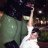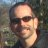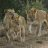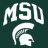# Premier Stochastic Oscillator

M

#### manishji

##### New member
any help getting pso... its calculated as below.

%K = 100 X [(C – Ln) / (Hn – Ln)]

Where

• C = the most recent closing price
• n = the lookback period
• Ln = the low of the n previous price bars
• Hn = the highest price during the same n period

The premier stochastic oscillator normalizes the standard stochastic oscillator by applying a five-period double exponential smoothing average of the %K value, resulting in a symmetric scale of 1 to -1. The PSO calculation, then, is:

PSO = (exponential value (S) – 1) / (exponential value (S) + 1)

Where

• S = 5-period double smoothed exponential EMA ((%K – 50) * .1)
• %K = 8-period stochastic oscillator#### horserider

##### Well-known member
VIP
This one ?

Code:
``````# // ==========================
# // PREMIER STOCHASTIC
# // Indicator
# // ==========================
#
# Technical Analysis of Stocks & Commodities
# August, 2008
# Premier Stochastic Oscillator by Lee Leibfarth
# pp 30 - 36
# ported by R Houser
#
declare lower;

# inputs:
input Line1 = 0.9;
input Line2 = 0.2;
input StochLength = 8;
input Period = 25;

# variables:
# oFastK(0),
# oFastD(0),
# oSlowK(0),
# oSlowD(0),
# Length(0),
# NormStoch(0),
# SmoothStoch(0),
# Premier(0);

# Value1 = Stochastic( h, l, c, StochLength, 1, 3, 1, oFastK, oFastD, oSlowK, oSlowD);
def oFastK = 100 * ( ( close - Lowest( low, StochLength ) ) / ( Highest( high, StochLength ) - Lowest( low, StochLength ) ) );
def oFastD = Average( oFastK, 1 );
def oSlowK = oFastD;
def oSlowD = Average( oSlowK, 3 );

# Length = iff(Period < 0, 1, squareroot(Period));
def Length = if Period < 0 then 1 else Sqrt( Period );

# NormStoch = .1 * (oslowK - 50);
def NormStoch = 0.1 * ( oSlowK - 50 );

# SmoothStoch = xaverage(xaverage(NormStoch, Length), Length);
def SmoothStoch = ExpAverage( ExpAverage( NormStoch, Length ), Length );

plot Premier = ( Exp( 1 * SmoothStoch ) - 1 ) / ( Exp( 1 * SmoothStoch ) + 1 );
Premier.SetDefaultColor( Color.BLUE );

plot pLine1 = Line1;
pLine1.SetDefaultColor( Color.BLACK );
plot pLine2 = Line2;
pLine2.SetDefaultColor( Color.GRAY );
plot nLine1 = -1 * Line1;
nLine1.SetDefaultColor( Color.BLACK );
plot nLine2 = -1 * Line2;
nLine2.SetDefaultColor( Color.GRAY );``````

•J007RMC, mc01439 and markos
M

#### manishji

##### New member#### horserider

##### Well-known member
VIP
@manishji The study was provided. Besides I do not see any particular advantage. The stochastic values can be changed to give what I believe are better signals than the Premier Stochastic. Try playing with stochastic and see what you can get.

Example of Premier vs stochastic with changed values.•markos, skynetgen and BenTen
M

#### manishji

##### New member
@horserider Thank you very much

T

##### Active member
2019 Donor
@horserider anyway of knowing the the exactly what exponential value is, thanks

This is the Premier Stochastics from the Stocks & Commodities August 6th 2008 By Lee Leibfarth, Does anyone you know exactly what exponential value is?#### horserider

##### Well-known member
VIP

•T

##### Active member
2019 Donor
Do you know exactly what exponential value is? for the above premier stochastic. I think it’s the 3 in the code could someone confirm.#### horserider

##### Well-known member
VIP
Here are the exponential calculations ;
def SmoothStoch = ExpAverage( ExpAverage( NormStoch, Length ), Length );
plot Premier = ( Exp( 1 * SmoothStoch ) - 1 ) / ( Exp( 1 * SmoothStoch ) + 1 );

I believe 3 is on of the stochastic lengths. Not sure, did not write it and do not use it.

•T

##### Active member
2019 Donor
Here are the exponential calculations ;
def SmoothStoch = ExpAverage( ExpAverage( NormStoch, Length ), Length );
plot Premier = ( Exp( 1 * SmoothStoch ) - 1 ) / ( Exp( 1 * SmoothStoch ) + 1 );

I believe 3 is on of the stochastic lengths. Not sure, did not write it and do not use it.

Thanks for taking the time. Was wondering if you could turn the Premier line into a histogram thats green above .2 and red below.2? thanks have a great weekend#### horserider

##### Well-known member
VIP
Ok Color coded line or remove the comment from front of this line of code if you prefer a histogram display.
#Premier.SetPaintingStrategy(PaintingStrategy.HISTOGRAM);

Code:
``````# // ==========================
# // PREMIER STOCHASTIC
# // Indicator
# // ==========================
#
# Technical Analysis of Stocks & Commodities
# August, 2008
# Premier Stochastic Oscillator by Lee Leibfarth
# pp 30 - 36
# ported by R Houser
#  Changed to histogram display by Horserider 10/11/219

declare lower;

# inputs:
input Line1 = 0.9;
input Line2 = 0.2;
input StochLength = 8;
input Period = 25;

# variables:
# oFastK(0),
# oFastD(0),
# oSlowK(0),
# oSlowD(0),
# Length(0),
# NormStoch(0),
# SmoothStoch(0),
# Premier(0);

# Value1 = Stochastic( h, l, c, StochLength, 1, 3, 1, oFastK, oFastD, oSlowK, oSlowD);
def oFastK = 100 * ( ( close - Lowest( low, StochLength ) ) / ( Highest( high, StochLength ) - Lowest( low, StochLength ) ) );
def oFastD = Average( oFastK, 1 );
def oSlowK = oFastD;
def oSlowD = Average( oSlowK, 3 );

# Length = iff(Period < 0, 1, squareroot(Period));
def Length = if Period < 0 then 1 else Sqrt( Period );

# NormStoch = .1 * (oslowK - 50);
def NormStoch = 0.1 * ( oSlowK - 50 );

# SmoothStoch = xaverage(xaverage(NormStoch, Length), Length);
def SmoothStoch = ExpAverage( ExpAverage( NormStoch, Length ), Length );

plot Premier = ( Exp( 1 * SmoothStoch ) - 1 ) / ( Exp( 1 * SmoothStoch ) + 1 );

plot pLine1 = Line1;
pLine1.SetDefaultColor( Color.BLACK );
plot pLine2 = Line2;
pLine2.SetDefaultColor( Color.GRAY );
plot nLine1 = -1 * Line1;
nLine1.SetDefaultColor( Color.BLACK );
plot nLine2 = -1 * Line2;
nLine2.SetDefaultColor( Color.GRAY );

#Premier.SetPaintingStrategy(PaintingStrategy.HISTOGRAM);
Premier.SetLineWeight(3);
Premier.DefineColor("Positive and Up", Color.GREEN);
Premier.DefineColor("Positive and Down", Color.DARK_GREEN);
Premier.DefineColor("Negative and Down", Color.RED);
Premier.DefineColor("Negative and Up", Color.DARK_RED);
Premier.AssignValueColor(if Premier >= 0 then if Premier >Premier then Premier.color("Positive and Up") else Premier.color("Positive and Down") else if Premier < Premier then Premier.color("Negative and Down") else Premier.color("Negative and Up"));``````

•M

#### Mlentsch0

##### New member
VIP
Example of Premier vs stochastic with changed values.what do you use for bar color?

T

##### Active member
2019 Donor
Ok Color coded line or remove the comment from front of this line of code if you prefer a histogram display.
#Premier.SetPaintingStrategy(PaintingStrategy.HISTOGRAM);

Code:
``````# // ==========================
# // PREMIER STOCHASTIC
# // Indicator
# // ==========================
#
# Technical Analysis of Stocks & Commodities
# August, 2008
# Premier Stochastic Oscillator by Lee Leibfarth
# pp 30 - 36
# ported by R Houser
#  Changed to histogram display by Horserider 10/11/219

declare lower;

# inputs:
input Line1 = 0.9;
input Line2 = 0.2;
input StochLength = 8;
input Period = 25;

# variables:
# oFastK(0),
# oFastD(0),
# oSlowK(0),
# oSlowD(0),
# Length(0),
# NormStoch(0),
# SmoothStoch(0),
# Premier(0);

# Value1 = Stochastic( h, l, c, StochLength, 1, 3, 1, oFastK, oFastD, oSlowK, oSlowD);
def oFastK = 100 * ( ( close - Lowest( low, StochLength ) ) / ( Highest( high, StochLength ) - Lowest( low, StochLength ) ) );
def oFastD = Average( oFastK, 1 );
def oSlowK = oFastD;
def oSlowD = Average( oSlowK, 3 );

# Length = iff(Period < 0, 1, squareroot(Period));
def Length = if Period < 0 then 1 else Sqrt( Period );

# NormStoch = .1 * (oslowK - 50);
def NormStoch = 0.1 * ( oSlowK - 50 );

# SmoothStoch = xaverage(xaverage(NormStoch, Length), Length);
def SmoothStoch = ExpAverage( ExpAverage( NormStoch, Length ), Length );

plot Premier = ( Exp( 1 * SmoothStoch ) - 1 ) / ( Exp( 1 * SmoothStoch ) + 1 );

plot pLine1 = Line1;
pLine1.SetDefaultColor( Color.BLACK );
plot pLine2 = Line2;
pLine2.SetDefaultColor( Color.GRAY );
plot nLine1 = -1 * Line1;
nLine1.SetDefaultColor( Color.BLACK );
plot nLine2 = -1 * Line2;
nLine2.SetDefaultColor( Color.GRAY );

#Premier.SetPaintingStrategy(PaintingStrategy.HISTOGRAM);
Premier.SetLineWeight(3);
Premier.DefineColor("Positive and Up", Color.GREEN);
Premier.DefineColor("Positive and Down", Color.DARK_GREEN);
Premier.DefineColor("Negative and Down", Color.RED);
Premier.DefineColor("Negative and Up", Color.DARK_RED);
Premier.AssignValueColor(if Premier >= 0 then if Premier >Premier then Premier.color("Positive and Up") else Premier.color("Positive and Down") else if Premier < Premier then Premier.color("Negative and Down") else Premier.color("Negative and Up"));``````
I have the code up but not sure what you mean remove the comment #Premier.SetPaintingStrategy(PaintingStrategy.HISTOGRAM);, not code savy so not sure what to do#### markos

##### Well-known member
VIP#### markos

##### Well-known member
VIP
I have the code up but not sure what you mean remove the comment #Premier.SetPaintingStrategy(PaintingStrategy.HISTOGRAM);, not code savy so not sure what to do
btw, a Comment is the pound sign # , just remove the sign from the line that was suggested. https://tlc.thinkorswim.com/center/reference/thinkScript.html#### horserider

##### Well-known member
VIP
what do you use for bar color?
Good question. I did that chart just to display the stochastic comparison. Erased after. Just looking at it looks like RSI colored candles.#### horserider

##### Well-known member
VIP
I have the code up but not sure what you mean remove the comment #Premier.SetPaintingStrategy(PaintingStrategy.HISTOGRAM);, not code savy so not sure what to do
Did you know you can also go into study settings and change "line" to "histogram.

•T

##### Active member
2019 Donor
Did you know you can also go into study settings and change "line" to "histogram
OK I went in and I tried to change "Line" to Histogram several times, I looked in TOS trying to find this small error and couldn't figure it out, i only spent the time on these two lines of code line 64 and 65, i tried a bunch of different ways, i looked at Constant and paint strategy and histogram area i see how it works but my adjustment of this is wrong
#Premier.SetPaintingStrategy PaintingStrategy.HISTOGRAM
Premier.SetLineWeight(3);
What line of code do I change "Line" from and add in "Histogram" ...Thanks#### horserider

##### Well-known member
VIP
Click on Studies. Click on GEAR icon on far right of the study of interest. Click on DRAW AS. Click on HISTOGRAM looking on.
APPLY

or

In the code;
#Premier.SetPaintingStrategy(PaintingStrategy.HISTOGRAM);
Change to;
Premier.SetPaintingStrategy(PaintingStrategy.HISTOGRAM);

•T

##### Active member
2019 Donor
So, Change to; was this missing piece I wasn’t even close into figuring that out..thanks for helping me learn

•markosModify Slow Stochastic to show signals within X bars Questions 6MACD and Stochastic with 3/8 ema Questions 25RSI Stochastic Strategy Questions 3Stochastic Scalper for ThinkorSwim Indicators 9